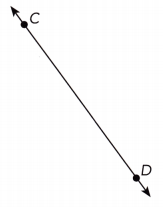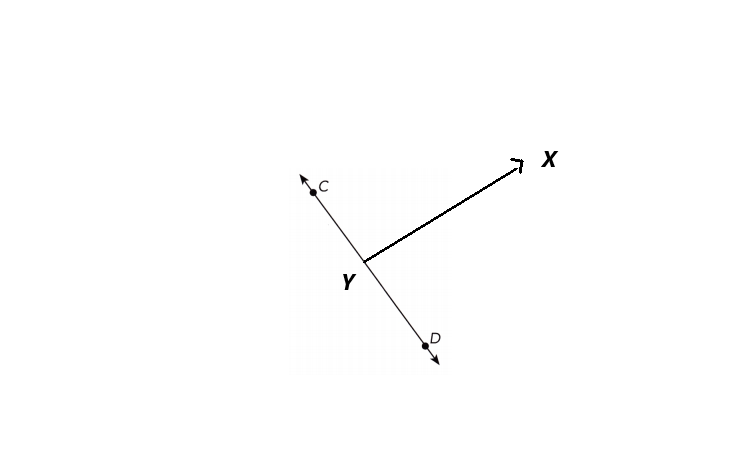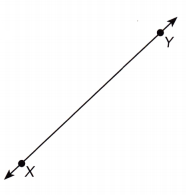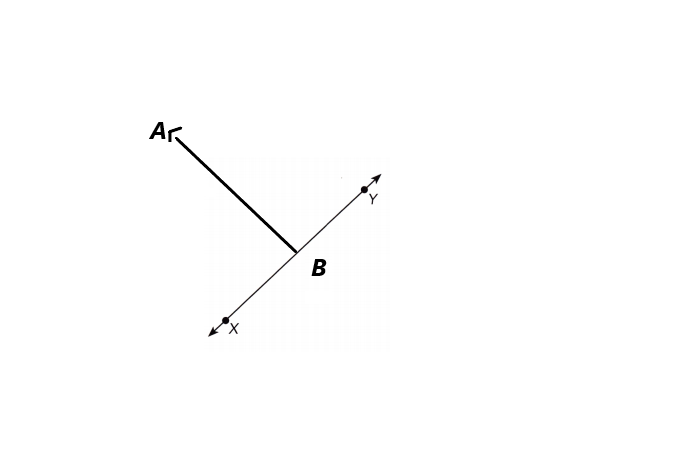# Math in Focus Grade 7 Chapter 7 Answer Key Geometric Construction

Practice the problems of Math in Focus Grade 7 Workbook Answer Key Chapter 7 Geometric Construction to score better marks in the exam.

## Math in Focus Grade 7 Course 2 B Chapter 7 Answer Key Geometric Construction

### Math in Focus Grade 7 Chapter 7 Quick Check Answer Key

Copy and complete the table by classifying the triangles.

Question 1.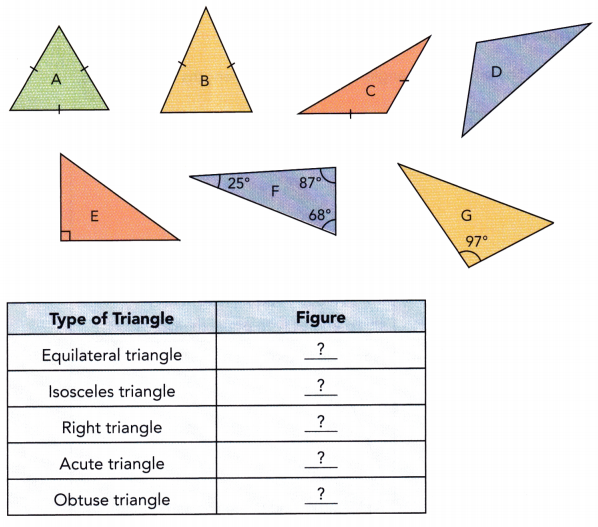Figure A is an Equilateral Triangle.
Figure B is an Isosceles Triangle.
Figure E is a Right Triangle.
Figure F is an Acute angle Triangle.
Figure C is an Obtuse angle Triangle

Explanation:

1. Equilateral triangle: In an equilateral triangle each angle is 60°. All sides and lengths of an equilateral triangle are equal. From the given figure we can classify that figure A is an Equilateral triangle.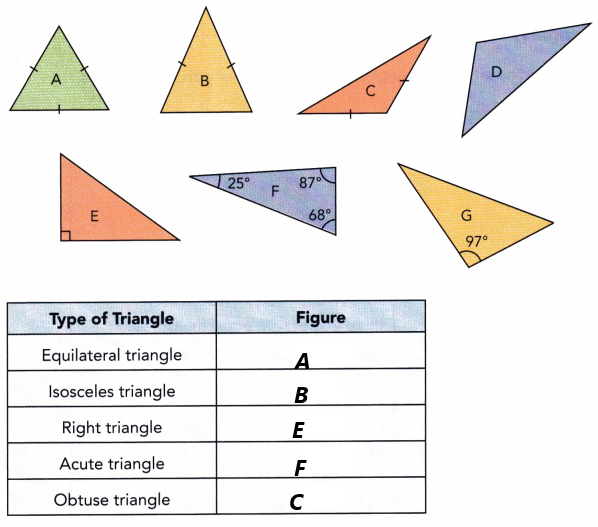2. Isosceles triangle: In the isosceles triangle only two sides are equal, the number of vertices and the number of edges are 3. By observing the given diagram figure B is called an Isosceles triangle
3. Right Triangle: A right-angled triangle is a triangle in which one of the angles is 90 degrees. Figure E is called a right-angled triangle.
4. Acute triangle: An acute angle is an angle that is less than 90. Since all the three angles are less than 90° then it is called an acute angle. Figure F is an acute angle from the above diagram.
5. Obtuse triangle: Obtuse angle is an angle that has greater than 90°. The figure C is called an obtuse triangle.

Copy and complete the table.

Question 2.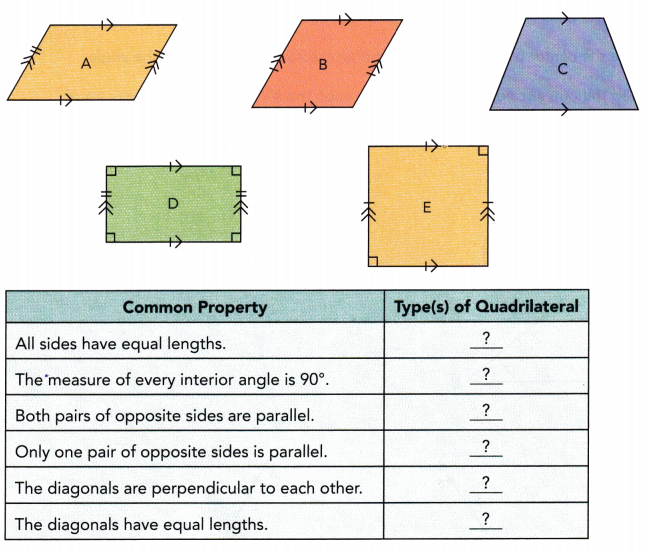1. Square: All sides are equal.
2. Rectangle: Rectangle is a quadrilateral with four right angles. The area of a rectangle is length width.
3.Trapezium: Trapezium has four vertices and four sides.
4. Parallelogram: In a parallelogram, both sides are parallel and equal.
5. Square parallelogram: The diagonals have equal length.

Explanation: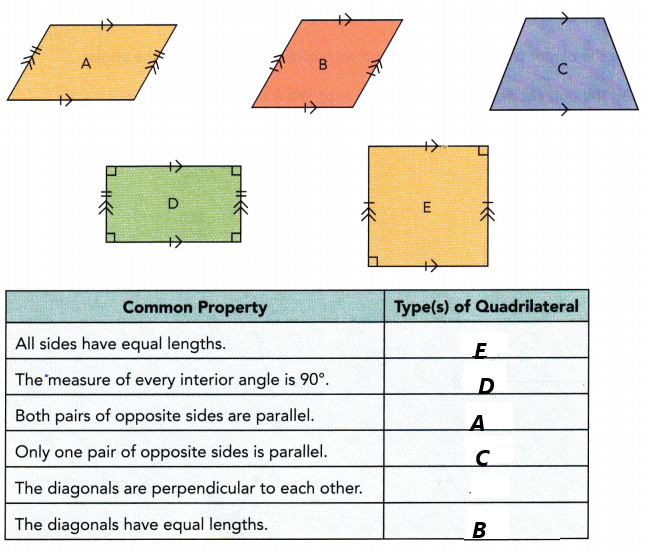Measure ∠ABC.

Question 3.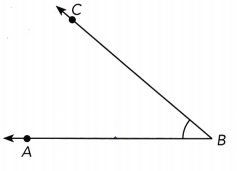∠ABC has 60° which is an acute angle triangle.

Explanation:
An acute angle is an angle that has less than 90°. The figure given here has less than 90° and the measure of that angle is 60°.

Question 4.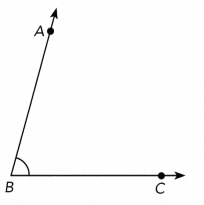The measurement of  ∠ABC is 80°. And it is an acute angle triangle.

Explanation:
An acute angle is an angle that has less than 90°. The figure given here has less than 90° and the measure of that angle is 80°.

Question 5.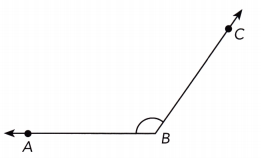The measurement of ∠ABC is 110°.

Explanation:
An obtuse angle is an angle that has greater than 90°. From the given figure we can measure the angle ∠ABC has 110°

Question 6.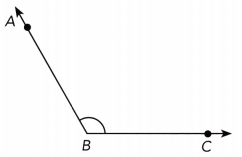The measurement of ∠ABC is 120°which is an obtuse angle triangle.

Explanation:
An obtuse angle is an angle that has greater than 90°. From the given figure we can measure the angle ∠ABC has 120°

Use a protractor to draw each angle.

Question 7.
m∠DEF = 39°
Angle DEF is an acute angle and the angle between 90° to 180°.

Explanation: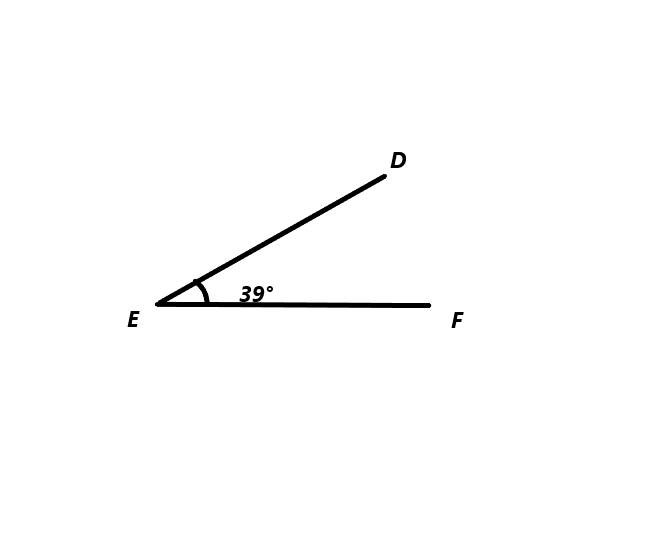Question 8.
m∠XYZ = 92°
Angle XYZ has a right angle and it is 92°.

Explanation: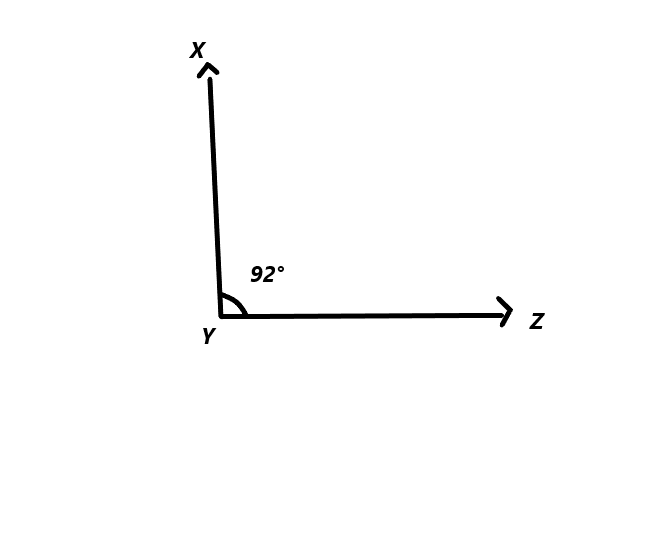Question 9.
m∠PQR = 146°
Angle PQR is 146°.

Explanation: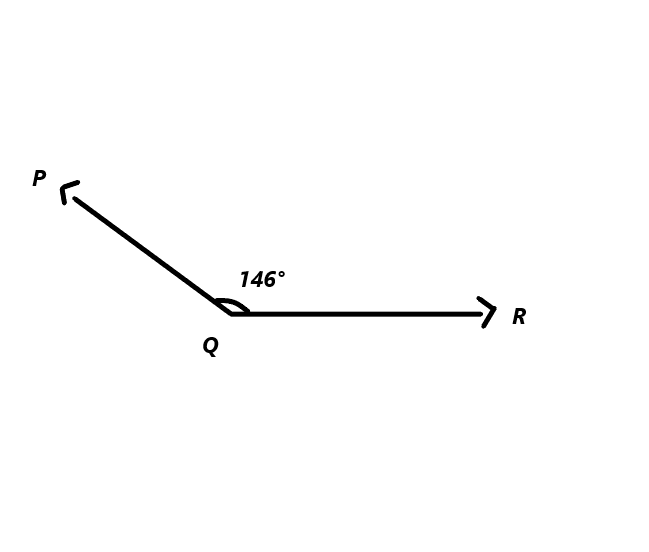Copy $$\overleftrightarrow{\mathbf{A B}}$$, $$\overleftrightarrow{\mathbf{C D}}$$, and $$\overleftrightarrow{\mathbf{X Y}}$$ on a sheet of paper. Draw a perpendicular line to each given line.

Question 10.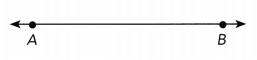We take the straight line AB  They insect at each at a right angle.

Explanation: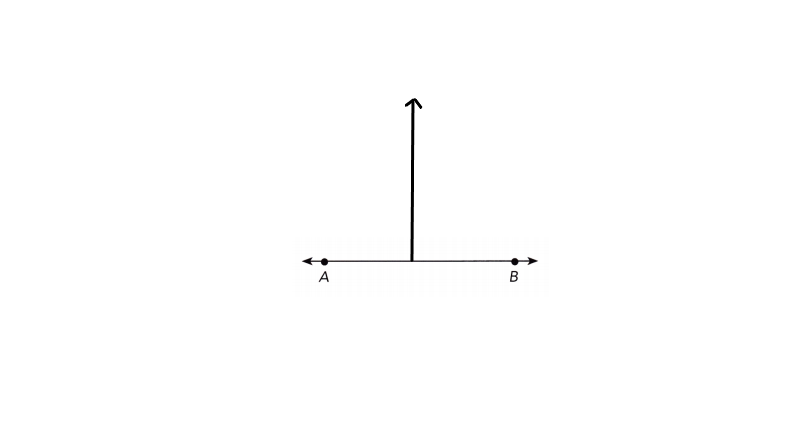Question 11.